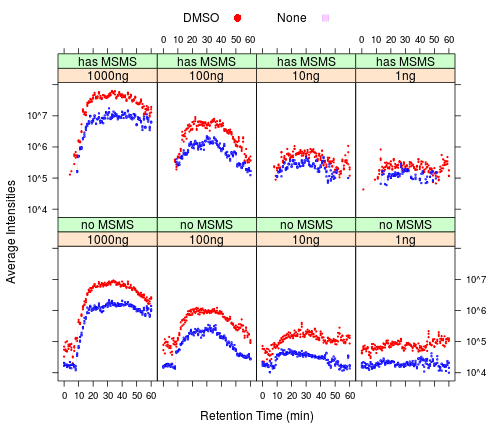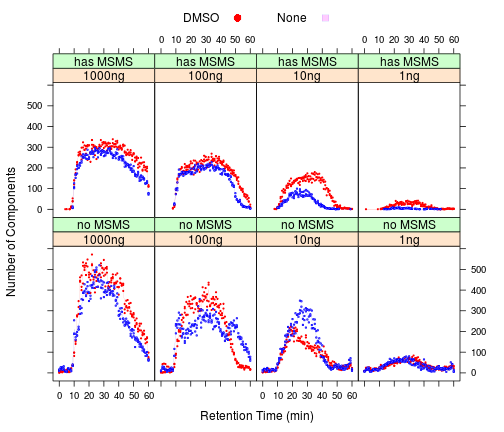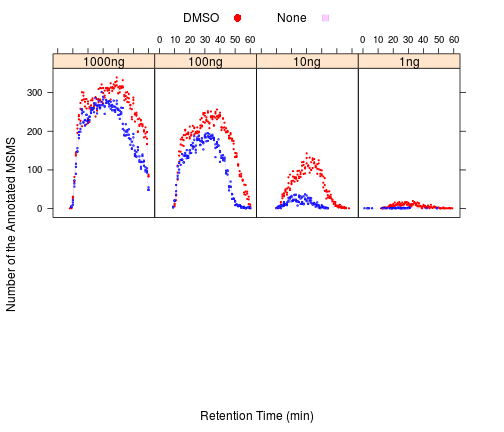# A Quick Look at the Dataset: “DMSO enhances electrospray response boosting sensitivity of proteomic experiments”

We report that low percentages of dimethylsulfoxide (DMSO) in liquid chromatography solvents lead to a strong enhancement of electrospray ionization of peptides, improving the sensitivity of protein identification in bottom up proteomics by up to tenfold. The method can be easily implemented on any LC-MS/MS system without modification to hardware or software and at no additional cost.

http://proteomecentral.proteomexchange.org/cgi/GetDataset?ID=PXD000254

``````## read the reports from the publication: xxxx
library(gtools)
library(lattice)
library(data.table)

# the place to find the data and store the results
dat.src = "/media/wyu/Padlock/PXD000254, DMSO boosts ESI sensitivity/reports/"

``````

### Data Inventory Tables

``````ftable(subset(annotated, sample == "HeLa")[, c("instrument", "additive", "loading")])
``````
``````##                     loading 1000ng 100ng  10ng   1ng unknown
## Elite      DMSO              39156 25692  8263   882   97801
##            None              31171 16367  1496    60   71586
## Velos      DMSO                  0     0     0     0   77187
##            None                  0     0     0     0   75622
## XL         DMSO                  0     0     0     0   60265
##            None                  0     0     0     0   60620
``````
``````ftable(subset(proteins, sample == "HeLa")[, c("instrument", "additive", "loading")])
``````
``````##                     loading 1000ng 100ng 10ng  1ng unknown
## Elite      DMSO               2320  1730  760  139    3013
##            None               1843  1303  244   29    2341
## Velos      DMSO                  0     0    0    0    2722
##            None                  0     0    0    0    2368
## XL         DMSO                  0     0    0    0    2613
##            None                  0     0    0    0    2224
``````
``````ftable(subset(components, sample == "HeLa")[, c("instrument", "additive", "loading")])
``````
``````##                     loading 1000ng  100ng   10ng    1ng unknown
## Elite      DMSO             103374  70365  36427  21393  175337
##            None              83868  59610  33447  13928  139954
## Velos      DMSO                  0      0      0      0  273277
##            None                  0      0      0      0  269492
## XL         DMSO                  0      0      0      0  230000
##            None                  0      0      0      0  216036
``````

### Significant advantages in LCMS components and peptide/protein identifications for the samples with DMSO

``````# prepare the aggregated stats
components\$Retention.time = as.numeric(as.character(components\$Retention.time))
components\$rtpost = round(components\$Retention.time)
components\$Intensity = as.numeric(as.character(components\$Intensity))
components\$MS.MS.Count = as.numeric(as.character(components\$MS.MS.Count))
components\$hasMSMS = NA
components[components\$MS.MS.Count > 0, ]\$hasMSMS = "has MSMS"
components[components\$MS.MS.Count == 0, ]\$hasMSMS = "no MSMS"

cmps.t = data.table(subset(components, Charge > 1))
cmps.s = cmps.t[, list(Counts = length(Intensity), Avg = mean(Intensity), MSMS = sum(MS.MS.Count)),
``````

### Intensities of the LCMS components are enhanced in the presence of DMSO

``````xyplot(Avg ~ rtpost | loading * hasMSMS, group = additive, data = cmps.s, subset = instrument ==
"Elite" & runtime == "1h", type = c("p", "a"), auto.key = list(space = "top",
columns = 2), layout = c(4, 2), as.table = TRUE, cex = 0.25, par.settings = simpleTheme(pch = c(19,
22), cex = 1.25, lwd = 0.1, col = c("red", "blue")), scale = list(y = list(relation = "same",
equispaced.log = TRUE, log = TRUE)), xlab = "Retention Time (min)", ylab = "Average Intensities")
``````### Though the number of the LCMS components remain about the same

``````xyplot(Counts ~ rtpost | loading * hasMSMS, group = additive, data = cmps.s,
subset = instrument == "Elite" & runtime == "1h", type = c("p", "a"), auto.key = list(space = "top",
columns = 2), layout = c(4, 2), as.table = TRUE, cex = 0.25, par.settings = simpleTheme(pch = c(19,
22), cex = 1.25, lwd = 0.1, col = c("red", "blue")), scale = list(y = list(relation = "same")),
xlab = "Retention Time (min)", ylab = "Number of Components")
````````````annotated\$Retention.time = as.numeric(as.character(annotated\$Retention.time))
annotated\$rtpost = round(annotated\$Retention.time)
annotated\$Mass.Error..ppm. = as.numeric(as.character(annotated\$Mass.Error..ppm.))
annotated\$Mass = as.numeric(as.character(annotated\$Mass))
annotated\$Chage = as.numeric(as.character(annotated\$Charge))

annt.t = data.table(annotated)
annt.s = annt.t[, list(Counts = length(Mass), AvgMass = mean(Mass), AvgZ = mean(Charge),
``````

### More MS/MS at higher %B with DMSO, hinting the its ability to prevent the loss of more hydrophobic peptides.

``````xyplot(Counts ~ rtpost | loading, group = additive, data = annt.s, subset = instrument ==
"Elite" & runtime == "1h", type = c("p", "a"), auto.key = list(space = "top",
columns = 2), layout = c(4, 2), as.table = TRUE, cex = 0.25, par.settings = simpleTheme(pch = c(19,
22), cex = 1.25, lwd = 0.1, col = c("red", "blue")), scale = list(y = list(relation = "same")),
xlab = "Retention Time (min)", ylab = "Number of the Annotated MSMS")
``````